Olympiad Test: Geometrical Shapes And Angles - 1

# Olympiad Test: Geometrical Shapes And Angles - 1

Test Description

## 10 Questions MCQ Test Math Olympiad for Class 5 | Olympiad Test: Geometrical Shapes And Angles - 1

Olympiad Test: Geometrical Shapes And Angles - 1 for Class 5 2023 is part of Math Olympiad for Class 5 preparation. The Olympiad Test: Geometrical Shapes And Angles - 1 questions and answers have been prepared according to the Class 5 exam syllabus.The Olympiad Test: Geometrical Shapes And Angles - 1 MCQs are made for Class 5 2023 Exam. Find important definitions, questions, notes, meanings, examples, exercises, MCQs and online tests for Olympiad Test: Geometrical Shapes And Angles - 1 below.
 1 Crore+ students have signed up on EduRev. Have you?
Olympiad Test: Geometrical Shapes And Angles - 1 - Question 1

### The angle 89° is:

Detailed Solution for Olympiad Test: Geometrical Shapes And Angles - 1 - Question 1

Since 89° is less than 90°, it is acute.

Olympiad Test: Geometrical Shapes And Angles - 1 - Question 2

### The angle 234° is:

Detailed Solution for Olympiad Test: Geometrical Shapes And Angles - 1 - Question 2

Since 234° is greater than 180°, it is reflex.

Olympiad Test: Geometrical Shapes And Angles - 1 - Question 3

### The angle 98° is:

Detailed Solution for Olympiad Test: Geometrical Shapes And Angles - 1 - Question 3

Since 98° is greater than 90° but less than 180°, it is obtuse

Olympiad Test: Geometrical Shapes And Angles - 1 - Question 4

Which is closest to the size of angle AOB?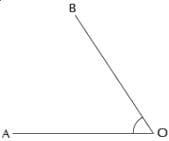Detailed Solution for Olympiad Test: Geometrical Shapes And Angles - 1 - Question 4

Measuring from O (Alphabet) in the direction of the arrow, angle AOB = 57°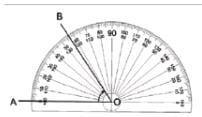Olympiad Test: Geometrical Shapes And Angles - 1 - Question 5

Which is closest to the size of angle COD?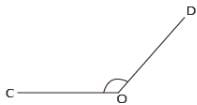Detailed Solution for Olympiad Test: Geometrical Shapes And Angles - 1 - Question 5

Starting from O, and measuring in the direction of the arrow, angle COD = 131°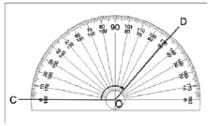Olympiad Test: Geometrical Shapes And Angles - 1 - Question 6

Which is closest to the size of reflex angle FOE?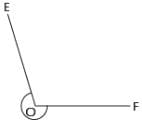Detailed Solution for Olympiad Test: Geometrical Shapes And Angles - 1 - Question 6

Reflex Angle FOE can be split into two:
Angle FOE = Angle FOG + Angle GOE
Angle FOG is a straight angle
∴ ∠FOG= 180°. Now, starting from
O and measuring in the direction of the arrow
Angle GOE = 74°
So Reflex Angle FOE = Angle FOG +
Angle GOE = 180° + 74° = 254°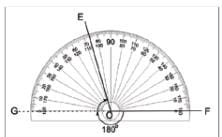Olympiad Test: Geometrical Shapes And Angles - 1 - Question 7

For the angle shown in the diagram, the arrow points to its :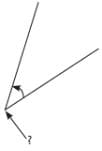Detailed Solution for Olympiad Test: Geometrical Shapes And Angles - 1 - Question 7

The vertex (plural vertices) is a corner point of two rays.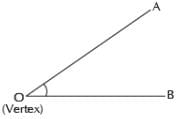Olympiad Test: Geometrical Shapes And Angles - 1 - Question 8

By using the three letters on the shape that define the angle, angle α is written as: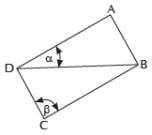Detailed Solution for Olympiad Test: Geometrical Shapes And Angles - 1 - Question 8

The middle letter is where the angle actually is (its vertex), which is D.
Therefore we could write the angle as ∠BDA or ∠ADB.

Olympiad Test: Geometrical Shapes And Angles - 1 - Question 9

By using the three letters on the shape that define the angle, angle b is written as: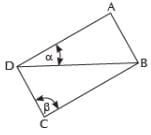Detailed Solution for Olympiad Test: Geometrical Shapes And Angles - 1 - Question 9

The middle letter is where the angle actually is (its vertex), which is C.
Therefore we could write the angle as ∠BCD or ∠DCB.

Olympiad Test: Geometrical Shapes And Angles - 1 - Question 10

If two acute angles are added together, which of the following is NOT possible for their sum:

Detailed Solution for Olympiad Test: Geometrical Shapes And Angles - 1 - Question 10

An acute angle is less than 90°.
If you add two acute angles then
(i) the sum could be acute, example: 10° + 20° = 30° (an acute angle).
(ii) the sum could be right, example: 40° + 50° = 90° (a right angle).
(iii) the sum could be obtuse, example: 30° + 80° = 110° (an obtuse angle).
(iv) the sum could NOT be straight because each acute angle is less than 90° and a straight angle is 180°, example: 89° + 89° = 178° which is less than a straight angle.

## Math Olympiad for Class 5

2 videos|3 docs|40 tests
 Use Code STAYHOME200 and get INR 200 additional OFF Use Coupon Code